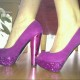# Mammal maths

1=A 11=K 21=U What is your favourite animal worth?
2=B 12=L 22=V What is the animal that is worth the highest?
3=C 13=M 23=W Can you find an animal that is worth 50?
4=D 14=N 24=X
5=E 15=O 25=Y
6=F 16=P 26=Z
7=G 17=Q
8=H 18=R
9=I 19=S
10=J 20=T

## 3 thoughts on “Mammal maths”

1.Creativity Mrs W says:

Thanks Jamie. I was thinking of this today. My favourite animals are frogs and monkeys so I am going to work this out 🙂

2.Rachel Orr says:

When I first read the title I thought it said ‘manimal maths’ and I was reminded of a television show about a character who could tranfORRm himself into an animal. The TV show was called ‘Manimal’. If you could be an animal, Jamie, what would you choose?

1.Jamie says:

I would be a Pygmy Seahorse and my mum would be a Rock hyrax.